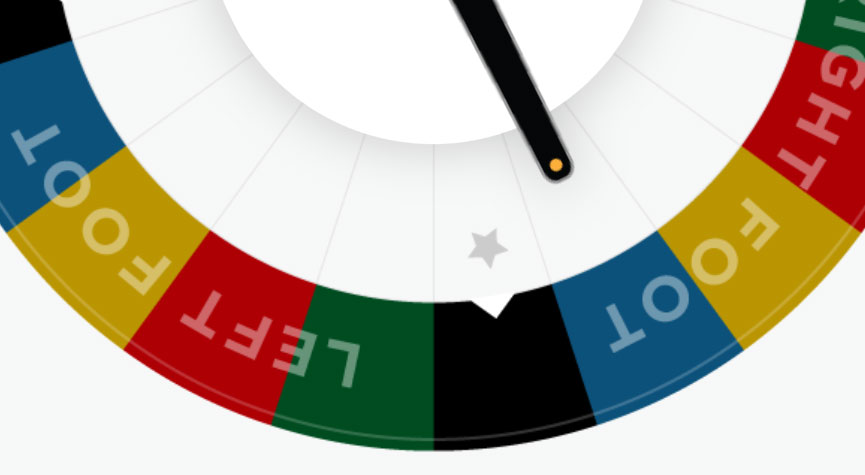# Getting the rotation angle after CABasicAnimation?I had a view that I rotate a lot, often more than 360 degrees (spins around a few times). Each time it stops, I wanted to determine the resulting “visual” angle. How does one go about doing that?

rotateView is a configured CABasicAnimation:
```let rotateView = CABasicAnimation()
let randonAngle = arc4random_uniform(361) + 1440
rotateView.fromValue = 0
rotateView.toValue = Float(randonAngle) * Float(M_PI) / Float(randomBetweenNumbers(firstNum: 90.0, secondNum: 180.0))//180.0
let randomSpeed = randomBetweenNumbers(firstNum: 1.5, secondNum: 2.1)
rotateView.duration = CFTimeInterval(randomSpeed)
rotateView.repeatCount = 0
rotateView.isRemovedOnCompletion = false
rotateView.fillMode = kCAFillModeForwards
rotateView.timingFunction = CAMediaTimingFunction(name: kCAMediaTimingFunctionEaseOut)

Once done, I dispatch after the randomSpeed duration so I know when the animation will complete. I then wanted the resulting angle. I searched all over the place & found a lot of old broken things and things that didn’t work for me.

This, however, does work (I spent an hour at the late night kitchen table trying things out)…

```let transform:CATransform3D = innerRing.layer.presentation()!.transform
let angle: CGFloat = atan2(transform.m12, transform.m11)
if testAngle < 0 {
testAngle = 360 + testAngle
}
print("final: \(testAngle)º")

// Here is the helper function